### IMO Shortlist 1987 problem 18

Kvaliteta:
Avg: 0,0
Težina:
Avg: 0,0
For any integer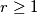$r \geq 1$, determine the smallest integer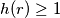$h(r) \geq 1$ such that for any partition of the set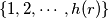$\{1, 2, \cdots, h(r)\}$ into$r$ classes, there are integers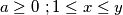$a \geq 0 \ ; 1 \leq x \leq y$, such that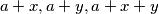$a + x, a + y, a + x + y$ belong to the same class.

Proposed by Romania
Izvor: Međunarodna matematička olimpijada, shortlist 1987